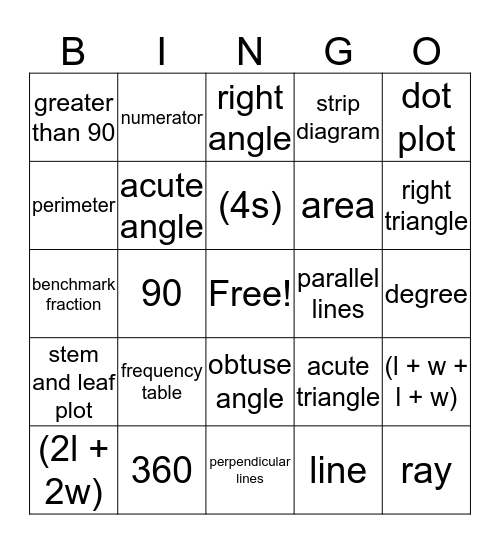# Math Vocabulary BingoThis bingo card has a free space and 30 words: 360, 180, 90, less than 90, greater than 90, right angle, acute angle, obtuse angle, parallel lines, perpendicular lines, degree, ray, line, line segment, right triangle, obtuse triangle, acute triangle, stem and leaf plot, frequency table, dot plot, benchmark fraction, denominator, numerator, area, perimeter, strip diagram, (l + w + l + w), (2l + 2w), (4s) and (l x w).

## Play Online